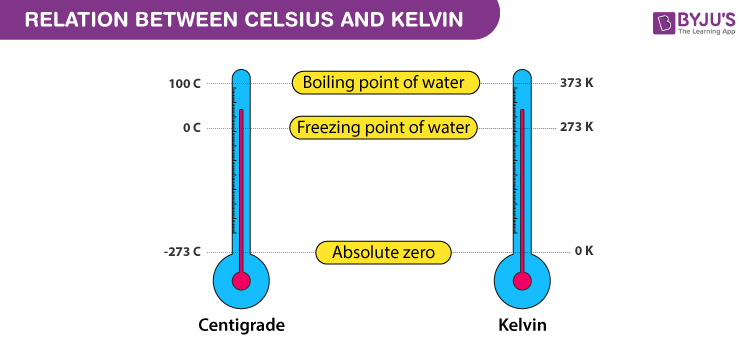# Relation Between Celsius And Kelvin

Celsius and Kelvin are two scales used to measure temperature. Both follow the same unit difference between each scale but with different starting points. The relation between Celsius and Kelvin are as follows-

## Celsius to Kelvin

 K = D+273

Where,

K is the temperature on the Kelvin scale.

D is the temperature on the Celsius scale.

## Kelvin to Celsius

 D = K – 273

Where,

K is the temperature on the Kelvin scale.

D is the temperature on the Celsius scale.

### Units of Celsius and Kelvin

• The temperature in the Celsius scale is denoted by 0C
• The temperature in the Kelvin scale is denoted by K

## Relationship between Celsius and KelvinBoth the scares are related as a unit for a unit. One unit increase in the Kelvin scale is the same as a one-degree increase in the Celsius scale. The only difference between these two scales is zero points of the thermometer.

Examples:

### 273 Kelvin to Celsius

D = K-273 ⇒ 273 – 273 00C

### 100 Celsius to Kelvin

K = D+273 ⇒ 100 + 273 373K

Hope you understood the relation and conversion between the Kelvin scale and the Celsius scale of temperature.

Physics Related Topics:

Stay tuned with BYJU’S for more such interesting articles. Also, register to “BYJU’S-The Learning App” for loads of interactive, engaging physics-related videos and an unlimited academic assist.

Test Your Knowledge On Relation Between Celsius And Kelvin!